Solutions and Colloids

# 67 Colligative Properties

### Learning Objectives

By the end of this section, you will be able to:

• Express concentrations of solution components using mole fraction and molality
• Describe the effect of solute concentration on various solution properties (vapor pressure, boiling point, freezing point, and osmotic pressure)
• Perform calculations using the mathematical equations that describe these various colligative effects
• Describe the process of distillation and its practical applications
• Explain the process of osmosis and describe how it is applied industrially and in nature

The properties of a solution are different from those of either the pure solute(s) or solvent. Many solution properties are dependent upon the chemical identity of the solute. Compared to pure water, a solution of hydrogen chloride is more acidic, a solution of ammonia is more basic, a solution of sodium chloride is more dense, and a solution of sucrose is more viscous. There are a few solution properties, however, that depend only upon the total concentration of solute species, regardless of their identities. These colligative properties include vapor pressure lowering, boiling point elevation, freezing point depression, and osmotic pressure. This small set of properties is of central importance to many natural phenomena and technological applications, as will be described in this module.

### Mole Fraction and Molality

Several units commonly used to express the concentrations of solution components were introduced in an earlier chapter of this text, each providing certain benefits for use in different applications. For example, molarity (M) is a convenient unit for use in stoichiometric calculations, since it is defined in terms of the molar amounts of solute species: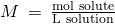Because solution volumes vary with temperature, molar concentrations will likewise vary. When expressed as molarity, the concentration of a solution with identical numbers of solute and solvent species will be different at different temperatures, due to the contraction/expansion of the solution. More appropriate for calculations involving many colligative properties are mole-based concentration units whose values are not dependent on temperature. Two such units are mole fraction (introduced in the previous chapter on gases) and molality.

The mole fraction, X, of a component is the ratio of its molar amount to the total number of moles of all solution components: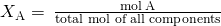By this definition, the sum of mole fractions for all solution components (the solvent and all solutes) is equal to one.

Molality is a concentration unit defined as the ratio of the numbers of moles of solute to the mass of the solvent in kilograms: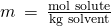Since these units are computed using only masses and molar amounts, they do not vary with temperature and, thus, are better suited for applications requiring temperature-independent concentrations, including several colligative properties, as will be described in this chapter module.

Calculating Mole Fraction and Molality The antifreeze in most automobile radiators is a mixture of equal volumes of ethylene glycol and water, with minor amounts of other additives that prevent corrosion. What are the (a) mole fraction and (b) molality of ethylene glycol, C2H4(OH)2, in a solution prepared from 2.22103 g of ethylene glycol and 2.00103 g of water (approximately 2 L of glycol and 2 L of water)?

Solution (a) The mole fraction of ethylene glycol may be computed by first deriving molar amounts of both solution components and then substituting these amounts into the definition of mole fraction.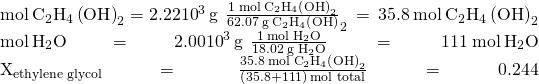Notice that mole fraction is a dimensionless property, being the ratio of properties with identical units (moles).

(b) Derive moles of solute and mass of solvent (in kg).

First, use the given mass of ethylene glycol and its molar mass to find the moles of solute: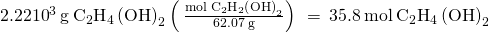Then, convert the mass of the water from grams to kilograms: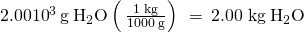Finally, calculate molarity per its definition:

*** QuickLaTeX cannot compile formula:
\begin{array}{}\\ \hfill \text{molality}& =& \phantom{\rule{0.2em}{0ex}}\frac{\text{mol solute}}{\text{kg solvent}}\hfill \\ \\ \hfill \text{molality}& =& \phantom{\rule{0.2em}{0ex}}\frac{35.8\phantom{\rule{0.2em}{0ex}}\text{mol}\phantom{\rule{0.2em}{0ex}}{\text{C}}_{2}{\text{H}}_{4}\left(\text{OH}{\right)}_{2}}{2\phantom{\rule{0.2em}{0ex}}\text{kg}\phantom{\rule{0.2em}{0ex}}{\text{H}}_{2}\text{O}}\hfill \\ \hfill \text{molality}& =& 17.9\phantom{\rule{0.2em}{0ex}}m\hfill \end{array}

*** Error message:
Missing # inserted in alignment preamble.
leading text: \begin{array}{} Extra alignment tab has been changed to \cr. leading text:\begin{array}{}\\ \hfill \text{molality}&
Extra alignment tab has been changed to \cr.
leading text: $\begin{array}{}\\ \hfill \text{molality}& =& Missing$ inserted.
leading text: ...\frac{\text{mol solute}}{\text{kg solvent}}
Extra }, or forgotten $. leading text: ...\frac{\text{mol solute}}{\text{kg solvent}} Missing } inserted. leading text: ...mol solute}}{\text{kg solvent}}\hfill \\ \\ Extra }, or forgotten$.
leading text: ...mol solute}}{\text{kg solvent}}\hfill \\ \\
Missing } inserted.
leading text: ...mol solute}}{\text{kg solvent}}\hfill \\ \\
Extra }, or forgotten . leading text: ...mol solute}}{\text{kg solvent}}\hfill \\ \\  Check Your Learning What are the mole fraction and molality of a solution that contains 0.850 g of ammonia, NH3, dissolved in 125 g of water? Answer: 7.1410−3; 0.399 m Converting Mole Fraction and Molal Concentrations Calculate the mole fraction of solute and solvent in a 3.0 m solution of sodium chloride. Solution Converting from one concentration unit to another is accomplished by first comparing the two unit definitions. In this case, both units have the same numerator (moles of solute) but different denominators. The provided molal concentration may be written as: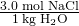The numerator for this solution’s mole fraction is, therefore, 3.0 mol NaCl. The denominator may be computed by deriving the molar amount of water corresponding to 1.0 kg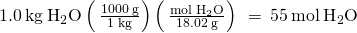and then substituting these molar amounts into the definition for mole fraction.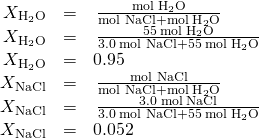Check Your Learning The mole fraction of iodine, I2, dissolved in dichloromethane, CH2Cl2, is 0.115. What is the molal concentration, m, of iodine in this solution? Answer: 1.50 m Molality and Molarity Conversions Intravenous infusion of a 0.556 M aqueous solution of glucose (density of 1.04 g/mL) is part of some post-operative recovery therapies. What is the molal concentration of glucose in this solution? Solution The provided molal concentration may be explicitly written as: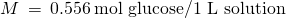Consider the definition of molality: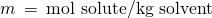The amount of glucose in 1-L of this solution is 0.556 mol, so the mass of water in this volume of solution is needed. First, compute the mass of 1.00 L of the solution: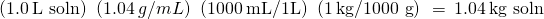This is the mass of both the water and its solute, glucose, and so the mass of glucose must be subtracted. Compute the mass of glucose from its molar amount: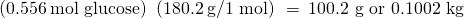Subtracting the mass of glucose yields the mass of water in the solution: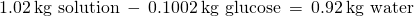Finally, the molality of glucose in this solution is computed as: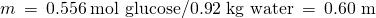Check Your Learning Nitric acid, HNO3(aq), is commercially available as a 33.7 m aqueous solution (density = 1.35 g/mL). What is the molarity of this solution? Answer: 14.6 M ### Vapor Pressure Lowering As described in the chapter on liquids and solids, the equilibrium vapor pressure of a liquid is the pressure exerted by its gaseous phase when vaporization and condensation are occurring at equal rates: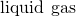Dissolving a nonvolatile substance in a volatile liquid results in a lowering of the liquid’s vapor pressure. This phenomenon can be rationalized by considering the effect of added solute molecules on the liquid’s vaporization and condensation processes. To vaporize, solvent molecules must be present at the surface of the solution. The presence of solute decreases the surface area available to solvent molecules and thereby reduces the rate of solvent vaporization. Since the rate of condensation is unaffected by the presence of solute, the net result is that the vaporization-condensation equilibrium is achieved with fewer solvent molecules in the vapor phase (i.e., at a lower vapor pressure) ((Figure)). While this interpretation is useful, it does not account for several important aspects of the colligative nature of vapor pressure lowering. A more rigorous explanation involves the property of entropy, a topic of discussion in a later text chapter on thermodynamics. For purposes of understanding the lowering of a liquid’s vapor pressure, it is adequate to note that the more dispersed nature of matter in a solution, compared to separate solvent and solute phases, serves to effectively stabilize the solvent molecules and hinder their vaporization. A lower vapor pressure results, and a correspondingly higher boiling point as described in the next section of this module. The presence of nonvolatile solutes lowers the vapor pressure of a solution by impeding the evaporation of solvent molecules.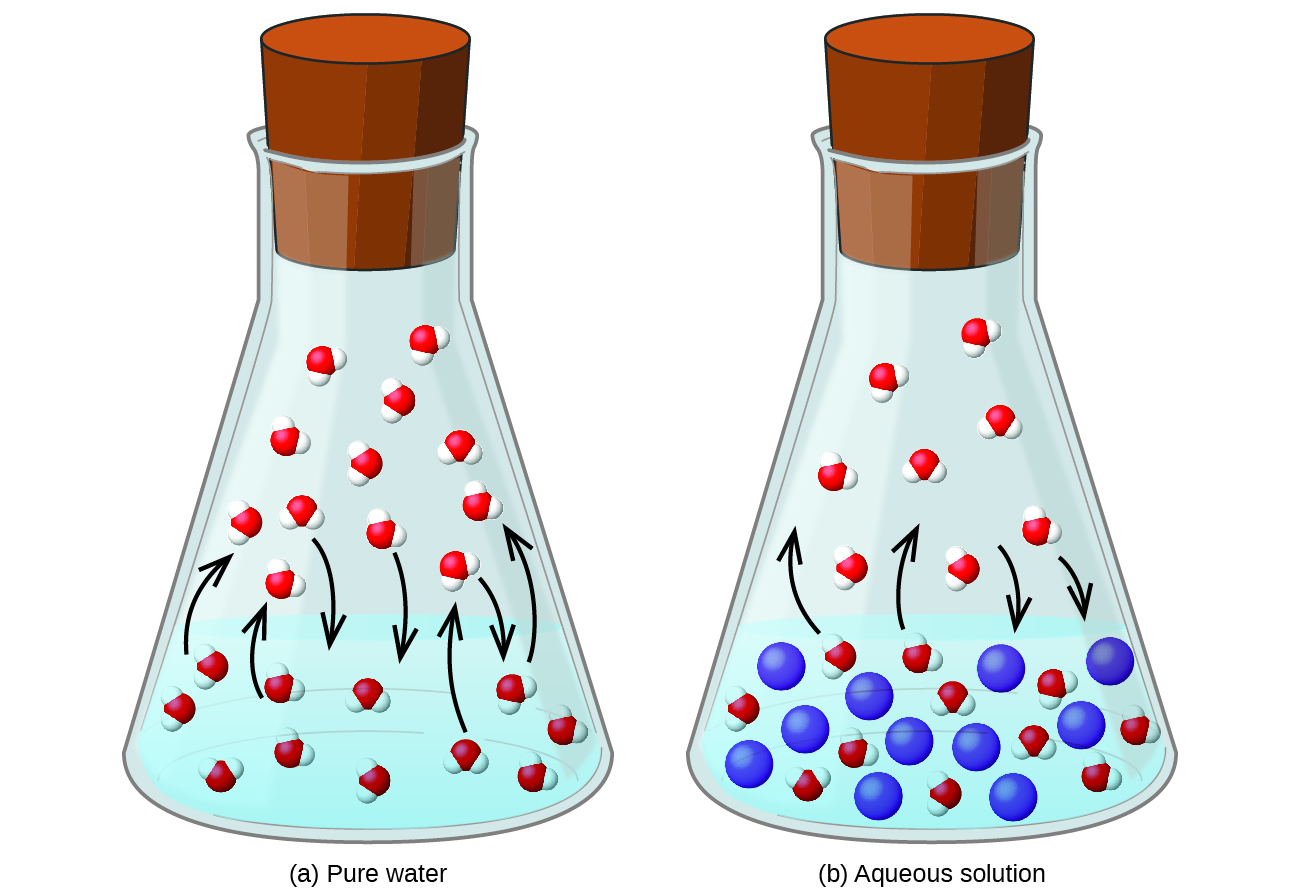The relationship between the vapor pressures of solution components and the concentrations of those components is described by Raoult’s law: The partial pressure exerted by any component of an ideal solution is equal to the vapor pressure of the pure component multiplied by its mole fraction in the solution.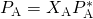where PA is the partial pressure exerted by component A in the solution,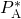is the vapor pressure of pure A, and XA is the mole fraction of A in the solution. Recalling that the total pressure of a gaseous mixture is equal to the sum of partial pressures for all its components (Dalton’s law of partial pressures), the total vapor pressure exerted by a solution containing i components is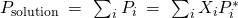A nonvolatile substance is one whose vapor pressure is negligible (P* ≈ 0), and so the vapor pressure above a solution containing only nonvolatile solutes is due only to the solvent: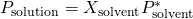Calculation of a Vapor Pressure Compute the vapor pressure of an ideal solution containing 92.1 g of glycerin, C3H5(OH)3, and 184.4 g of ethanol, C2H5OH, at 40 °C. The vapor pressure of pure ethanol is 0.178 atm at 40 °C. Glycerin is essentially nonvolatile at this temperature. Solution Since the solvent is the only volatile component of this solution, its vapor pressure may be computed per Raoult’s law as:First, calculate the molar amounts of each solution component using the provided mass data.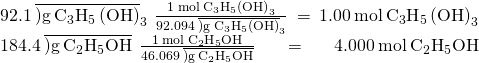Next, calculate the mole fraction of the solvent (ethanol) and use Raoult’s law to compute the solution’s vapor pressure.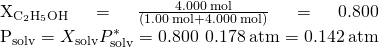Check Your Learning A solution contains 5.00 g of urea, CO(NH2)2 (a nonvolatile solute) and 0.100 kg of water. If the vapor pressure of pure water at 25 °C is 23.7 torr, what is the vapor pressure of the solution assuming ideal behavior? Answer: 23.4 torr ### Distillation of Solutions Solutions whose components have significantly different vapor pressures may be separated by a selective vaporization process known as distillation. Consider the simple case of a mixture of two volatile liquids, A and B, with A being the more volatile liquid. Raoult’s law can be used to show that the vapor above the solution is enriched in component A, that is, the mole fraction of A in the vapor is greater than the mole fraction of A in the liquid (see end-of-chapter Exercise 65). By appropriately heating the mixture, component A may be vaporized, condensed, and collected—effectively separating it from component B. Distillation is widely applied in both laboratory and industrial settings, being used to refine petroleum, to isolate fermentation products, and to purify water. A typical apparatus for laboratory-scale distillations is shown in (Figure). A typical laboratory distillation unit is shown in (a) a photograph and (b) a schematic diagram of the components. (credit a: modification of work by “Rifleman82”/Wikimedia commons; credit b: modification of work by “Slashme”/Wikimedia Commons)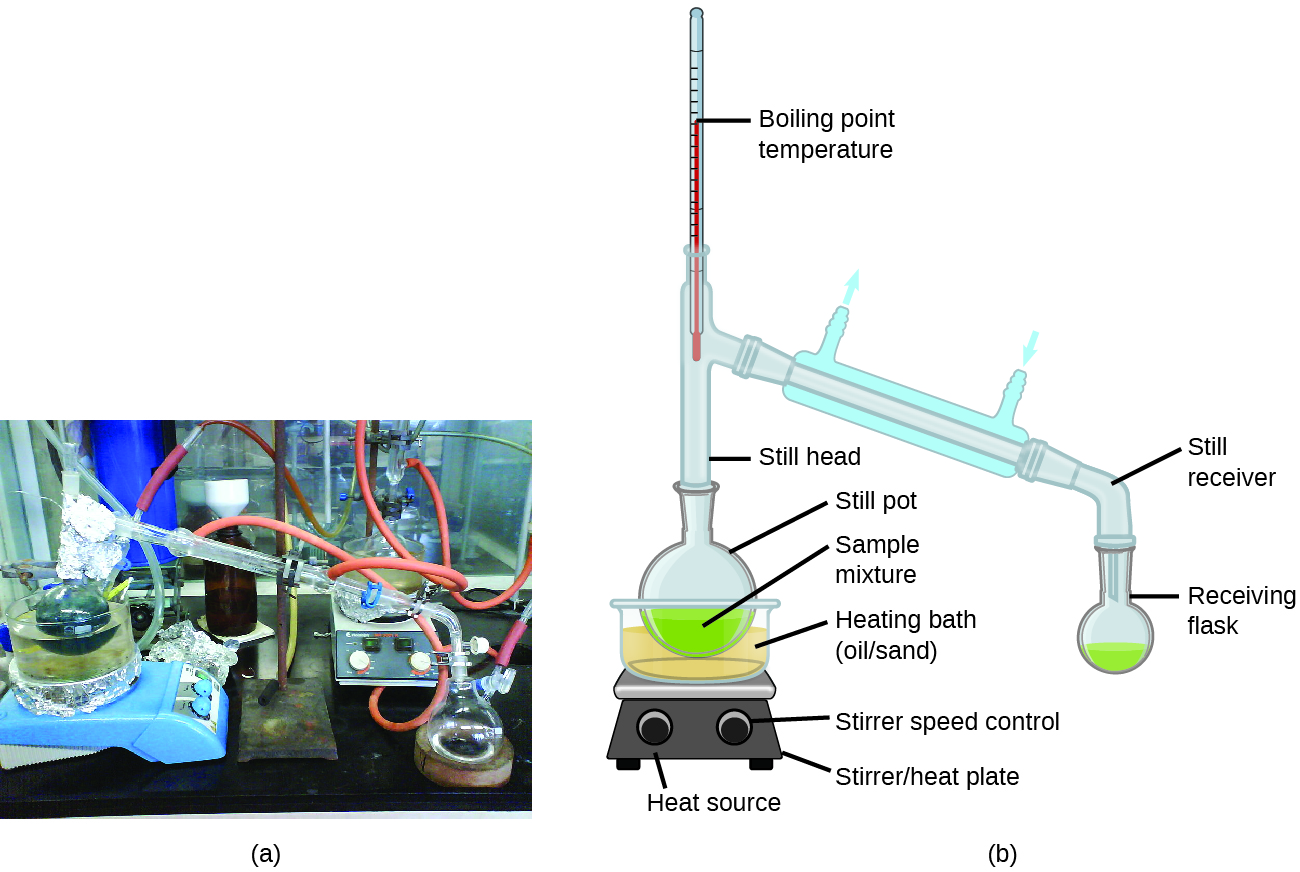Oil refineries use large-scale fractional distillation to separate the components of crude oil. The crude oil is heated to high temperatures at the base of a tall fractionating column, vaporizing many of the components that rise within the column. As vaporized components reach adequately cool zones during their ascent, they condense and are collected. The collected liquids are simpler mixtures of hydrocarbons and other petroleum compounds that are of appropriate composition for various applications (e.g., diesel fuel, kerosene, gasoline), as depicted in (Figure). Crude oil is a complex mixture that is separated by large-scale fractional distillation to isolate various simpler mixtures.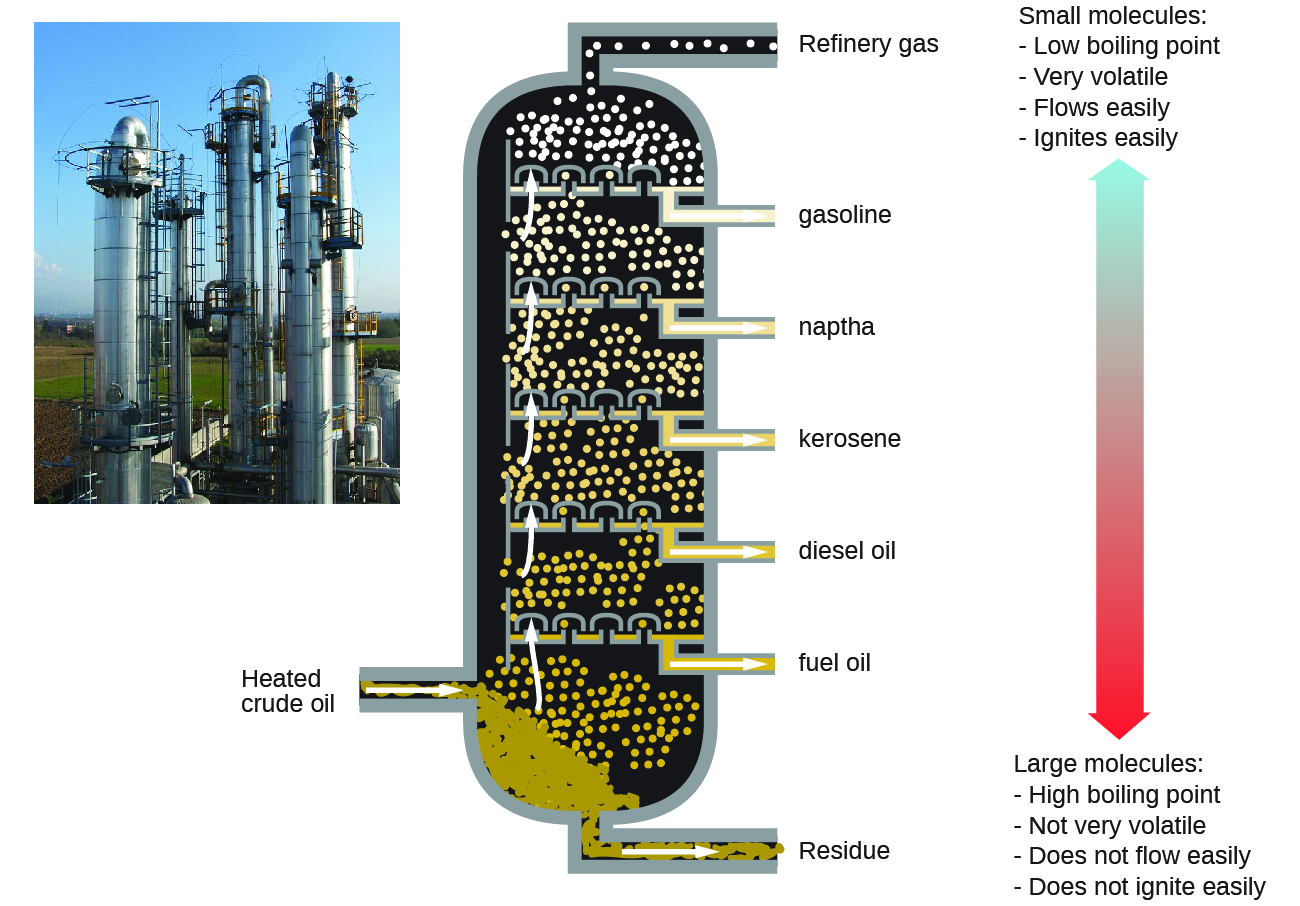### Boiling Point Elevation As described in the chapter on liquids and solids, the boiling point of a liquid is the temperature at which its vapor pressure is equal to ambient atmospheric pressure. Since the vapor pressure of a solution is lowered due to the presence of nonvolatile solutes, it stands to reason that the solution’s boiling point will subsequently be increased. Vapor pressure increases with temperature, and so a solution will require a higher temperature than will pure solvent to achieve any given vapor pressure, including one equivalent to that of the surrounding atmosphere. The increase in boiling point observed when nonvolatile solute is dissolved in a solvent, ΔTb, is called boiling point elevation and is directly proportional to the molal concentration of solute species: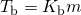where Kb is the boiling point elevation constant, or the ebullioscopic constant and m is the molal concentration (molality) of all solute species. Boiling point elevation constants are characteristic properties that depend on the identity of the solvent. Values of Kb for several solvents are listed in (Figure). Boiling Point Elevation and Freezing Point Depression Constants for Several Solvents Solvent Boiling Point (°C at 1 atm) Kb (ºCm−1) Freezing Point (°C at 1 atm) Kf (ºCm−1) water 100.0 0.512 0.0 1.86 hydrogen acetate 118.1 3.07 16.6 3.9 benzene 80.1 2.53 5.5 5.12 chloroform 61.26 3.63 −63.5 4.68 nitrobenzene 210.9 5.24 5.67 8.1 The extent to which the vapor pressure of a solvent is lowered and the boiling point is elevated depends on the total number of solute particles present in a given amount of solvent, not on the mass or size or chemical identities of the particles. A 1 m aqueous solution of sucrose (342 g/mol) and a 1 m aqueous solution of ethylene glycol (62 g/mol) will exhibit the same boiling point because each solution has one mole of solute particles (molecules) per kilogram of solvent. Calculating the Boiling Point of a Solution Assuming ideal solution behavior, what is the boiling point of a 0.33 m solution of a nonvolatile solute in benzene? Solution Use the equation relating boiling point elevation to solute molality to solve this problem in two steps.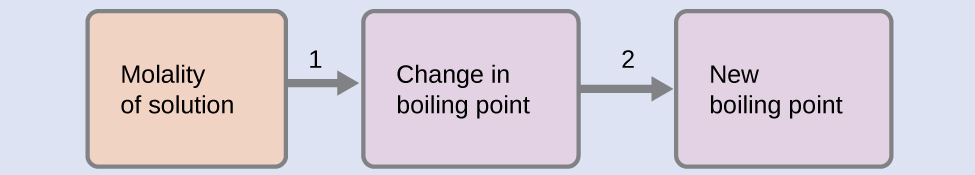1. Calculate the change in boiling point.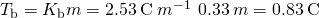2. Add the boiling point elevation to the pure solvent’s boiling point.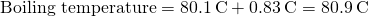Check Your LearningAssuming ideal solution behavior, what is the boiling point of the antifreeze described in (Figure)? Answer: 109.2 °C The Boiling Point of an Iodine Solution Find the boiling point of a solution of 92.1 g of iodine, I2, in 800.0 g of chloroform, CHCl3, assuming that the iodine is nonvolatile and that the solution is ideal. Solution A four-step approach to solving this problem is outlined below.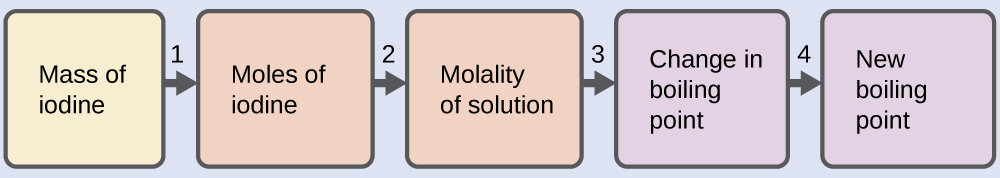1. Convert from grams to moles of I2using the molar mass of I2in the unit conversion factor. Result: 0.363 mol 2. Determine the molality of the solution from the number of moles of solute and the mass of solvent, in kilograms. Result: 0.454 m 3. Use the direct proportionality between the change in boiling point and molal concentration to determine how much the boiling point changes. Result: 1.65 °C 4. Determine the new boiling point from the boiling point of the pure solvent and the change. Result: 62.91 °C Check each result as a self-assessment. Check Your Learning What is the boiling point of a solution of 1.0 g of glycerin, C3H5(OH)3, in 47.8 g of water? Assume an ideal solution. Answer: 100.12 °C ### Freezing Point Depression Solutions freeze at lower temperatures than pure liquids. This phenomenon is exploited in “de-icing” schemes that use salt ((Figure)), calcium chloride, or urea to melt ice on roads and sidewalks, and in the use of ethylene glycol as an “antifreeze” in automobile radiators. Seawater freezes at a lower temperature than fresh water, and so the Arctic and Antarctic oceans remain unfrozen even at temperatures below 0 °C (as do the body fluids of fish and other cold-blooded sea animals that live in these oceans). Rock salt (NaCl), calcium chloride (CaCl2), or a mixture of the two are used to melt ice. (credit: modification of work by Eddie Welker)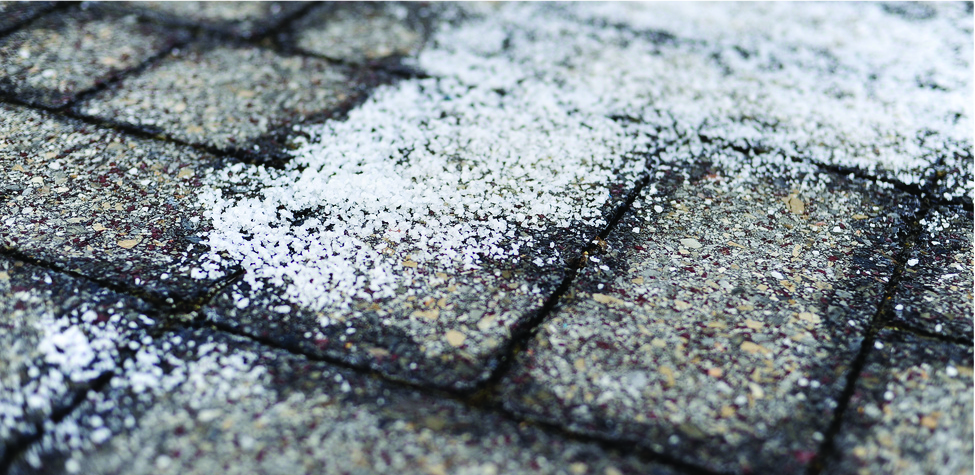The decrease in freezing point of a dilute solution compared to that of the pure solvent, ΔTf, is called the freezing point depression and is directly proportional to the molal concentration of the solute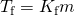where m is the molal concentration of the solute and Kf is called the freezing point depression constant (or cryoscopic constant). Just as for boiling point elevation constants, these are characteristic properties whose values depend on the chemical identity of the solvent. Values of Kf for several solvents are listed in (Figure). Calculation of the Freezing Point of a SolutionAssuming ideal solution behavior, what is the freezing point of the 0.33 m solution of a nonvolatile nonelectrolyte solute in benzene described in (Figure)? Solution Use the equation relating freezing point depression to solute molality to solve this problem in two steps.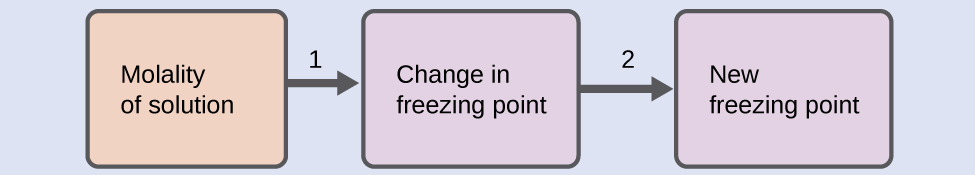1. Calculate the change in freezing point.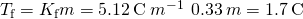2. Subtract the freezing point change observed from the pure solvent’s freezing point.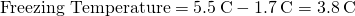Check Your Learning Assuming ideal solution behavior, what is the freezing point of a 1.85 m solution of a nonvolatile nonelectrolyte solute in nitrobenzene? Answer: −9.3 °C Colligative Properties and De-Icing Sodium chloride and its group 2 analogs calcium and magnesium chloride are often used to de-ice roadways and sidewalks, due to the fact that a solution of any one of these salts will have a freezing point lower than 0 °C, the freezing point of pure water. The group 2 metal salts are frequently mixed with the cheaper and more readily available sodium chloride (“rock salt”) for use on roads, since they tend to be somewhat less corrosive than the NaCl, and they provide a larger depression of the freezing point, since they dissociate to yield three particles per formula unit, rather than two particles like the sodium chloride. Because these ionic compounds tend to hasten the corrosion of metal, they would not be a wise choice to use in antifreeze for the radiator in your car or to de-ice a plane prior to takeoff. For these applications, covalent compounds, such as ethylene or propylene glycol, are often used. The glycols used in radiator fluid not only lower the freezing point of the liquid, but they elevate the boiling point, making the fluid useful in both winter and summer. Heated glycols are often sprayed onto the surface of airplanes prior to takeoff in inclement weather in the winter to remove ice that has already formed and prevent the formation of more ice, which would be particularly dangerous if formed on the control surfaces of the aircraft ((Figure)). Freezing point depression is exploited to remove ice from (a) roadways and (b) the control surfaces of aircraft.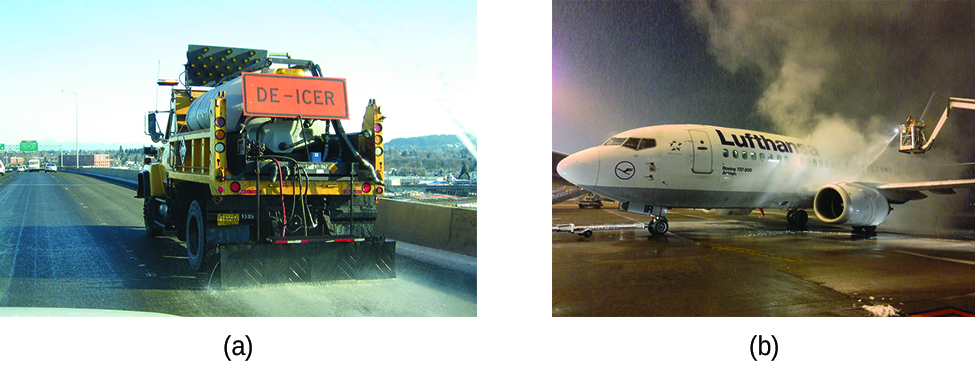### Phase Diagram for a Solution The colligative effects on vapor pressure, boiling point, and freezing point described in the previous section are conveniently summarized by comparing the phase diagrams for a pure liquid and a solution derived from that liquid ((Figure)). Phase diagrams for a pure solvent (solid curves) and a solution formed by dissolving nonvolatile solute in the solvent (dashed curves).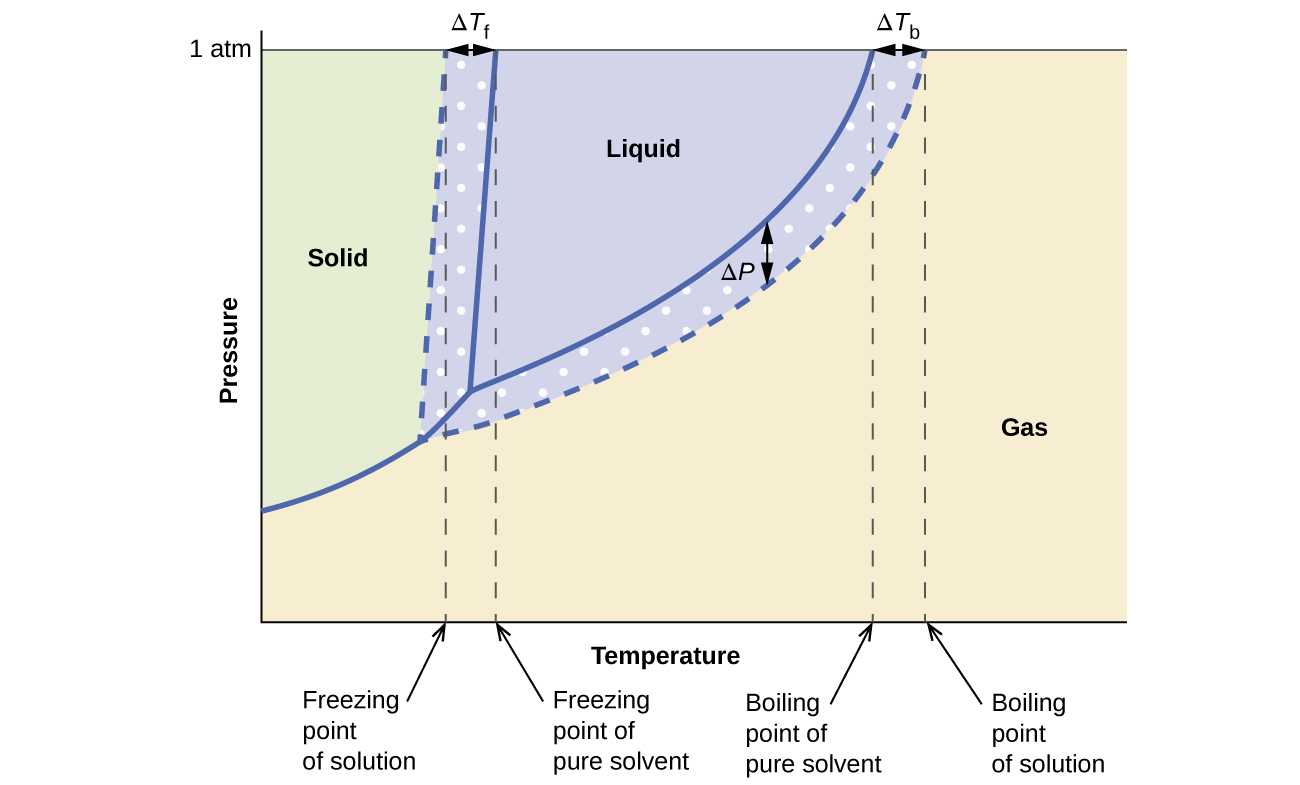The liquid-vapor curve for the solution is located beneath the corresponding curve for the solvent, depicting the vapor pressure lowering, ΔP, that results from the dissolution of nonvolatile solute. Consequently, at any given pressure, the solution’s boiling point is observed at a higher temperature than that for the pure solvent, reflecting the boiling point elevation, ΔTb, associated with the presence of nonvolatile solute. The solid-liquid curve for the solution is displaced left of that for the pure solvent, representing the freezing point depression, ΔTf, that accompanies solution formation. Finally, notice that the solid-gas curves for the solvent and its solution are identical. This is the case for many solutions comprising liquid solvents and nonvolatile solutes. Just as for vaporization, when a solution of this sort is frozen, it is actually just the solvent molecules that undergo the liquid-to-solid transition, forming pure solid solvent that excludes solute species. The solid and gaseous phases, therefore, are composed of solvent only, and so transitions between these phases are not subject to colligative effects. ### Osmosis and Osmotic Pressure of Solutions A number of natural and synthetic materials exhibit selective permeation, meaning that only molecules or ions of a certain size, shape, polarity, charge, and so forth, are capable of passing through (permeating) the material. Biological cell membranes provide elegant examples of selective permeation in nature, while dialysis tubing used to remove metabolic wastes from blood is a more simplistic technological example. Regardless of how they may be fabricated, these materials are generally referred to as semipermeable membranes. Consider the apparatus illustrated in (Figure), in which samples of pure solvent and a solution are separated by a membrane that only solvent molecules may permeate. Solvent molecules will diffuse across the membrane in both directions. Since the concentration of solvent is greater in the pure solvent than the solution, these molecules will diffuse from the solvent side of the membrane to the solution side at a faster rate than they will in the reverse direction. The result is a net transfer of solvent molecules from the pure solvent to the solution. Diffusion-driven transfer of solvent molecules through a semipermeable membrane is a process known as osmosis. (a) A solution and pure solvent are initially separated by an osmotic membrane. (b) Net transfer of solvent molecules to the solution occurs until its osmotic pressure yields equal rates of transfer in both directions.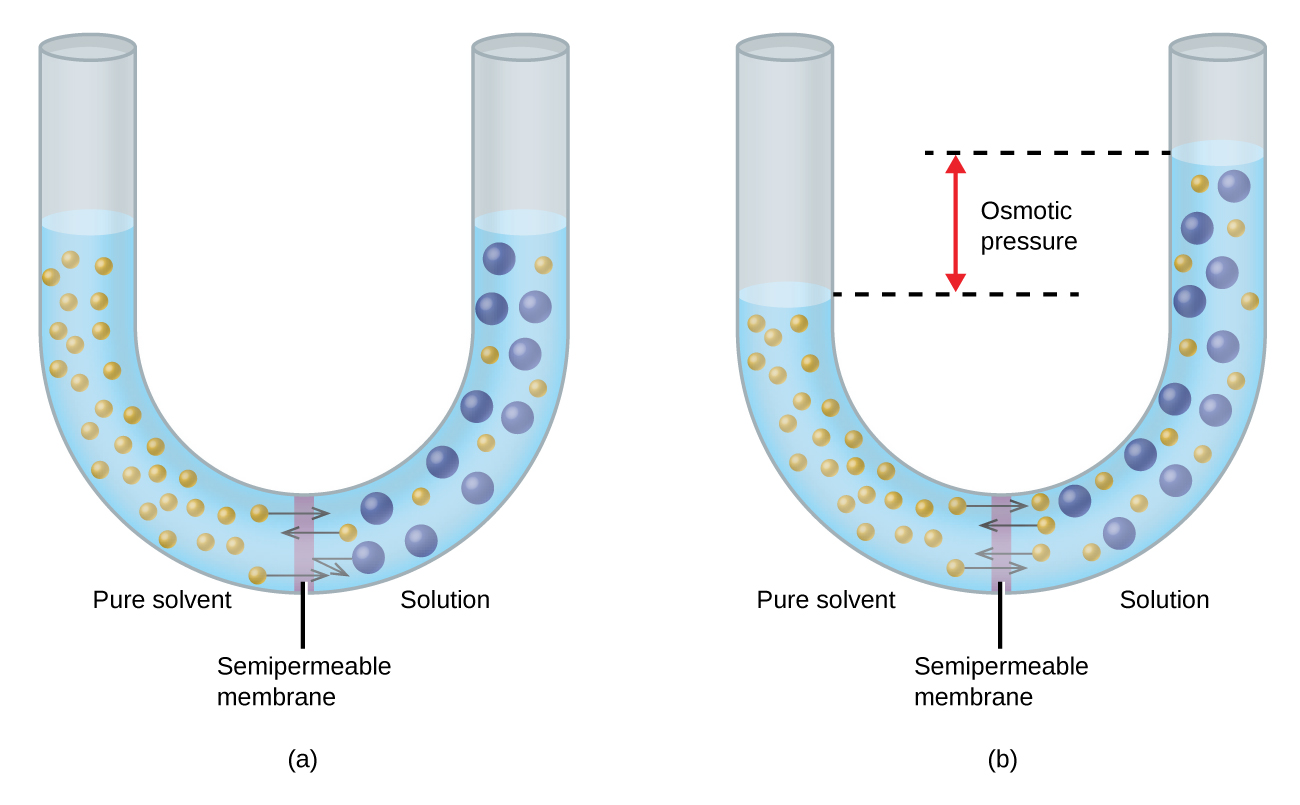When osmosis is carried out in an apparatus like that shown in (Figure), the volume of the solution increases as it becomes diluted by accumulation of solvent. This causes the level of the solution to rise, increasing its hydrostatic pressure (due to the weight of the column of solution in the tube) and resulting in a faster transfer of solvent molecules back to the pure solvent side. When the pressure reaches a value that yields a reverse solvent transfer rate equal to the osmosis rate, bulk transfer of solvent ceases. This pressure is called the osmotic pressure (Π) of the solution. The osmotic pressure of a dilute solution is related to its solute molarity, M, and absolute temperature, T, according to the equation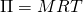where R is the universal gas constant. Calculation of Osmotic Pressure Assuming ideal solution behavior, what is the osmotic pressure (atm) of a 0.30 M solution of glucose in water that is used for intravenous infusion at body temperature, 37 °C? Solution Find the osmotic pressure, Π, using the formula Π = MRT, where T is on the Kelvin scale (310 K) and the value of R is expressed in appropriate units (0.08206 L atm/mol K).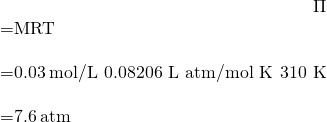Check Your Learning Assuming ideal solution behavior, what is the osmotic pressure (atm) a solution with a volume of 0.750 L that contains 5.0 g of methanol, CH3OH, in water at 37 °C? Answer: 5.3 atm If a solution is placed in an apparatus like the one shown in (Figure), applying pressure greater than the osmotic pressure of the solution reverses the osmosis and pushes solvent molecules from the solution into the pure solvent. This technique of reverse osmosis is used for large-scale desalination of seawater and on smaller scales to produce high-purity tap water for drinking. Applying a pressure greater than the osmotic pressure of a solution will reverse osmosis. Solvent molecules from the solution are pushed into the pure solvent.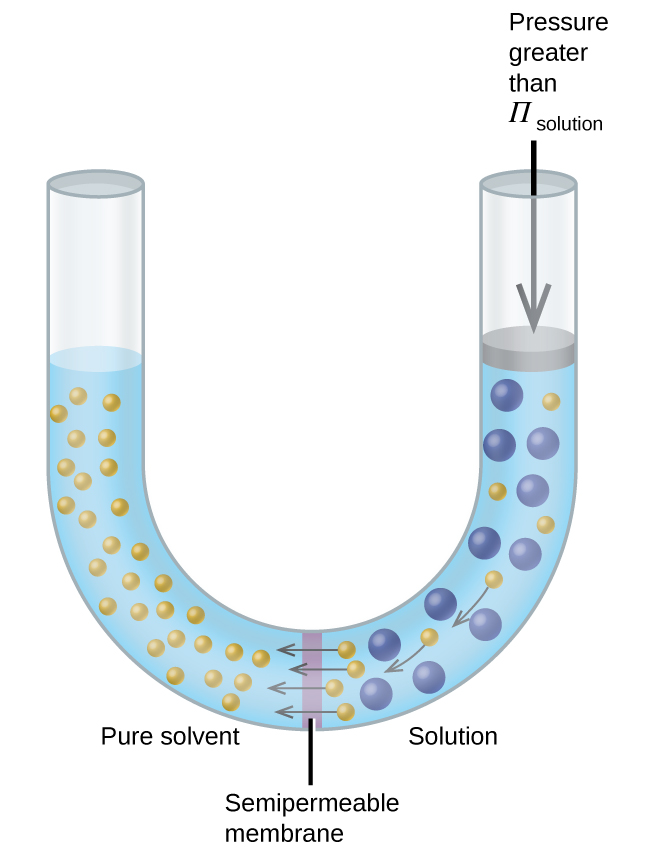Reverse Osmosis Water Purification In the process of osmosis, diffusion serves to move water through a semipermeable membrane from a less concentrated solution to a more concentrated solution. Osmotic pressure is the amount of pressure that must be applied to the more concentrated solution to cause osmosis to stop. If greater pressure is applied, the water will go from the more concentrated solution to a less concentrated (more pure) solution. This is called reverse osmosis. Reverse osmosis (RO) is used to purify water in many applications, from desalination plants in coastal cities, to water-purifying machines in grocery stores ((Figure)), and smaller reverse-osmosis household units. With a hand-operated pump, small RO units can be used in third-world countries, disaster areas, and in lifeboats. Our military forces have a variety of generator-operated RO units that can be transported in vehicles to remote locations. Reverse osmosis systems for purifying drinking water are shown here on (a) small and (b) large scales. (credit a: modification of work by Jerry Kirkhart; credit b: modification of work by Willard J. Lathrop)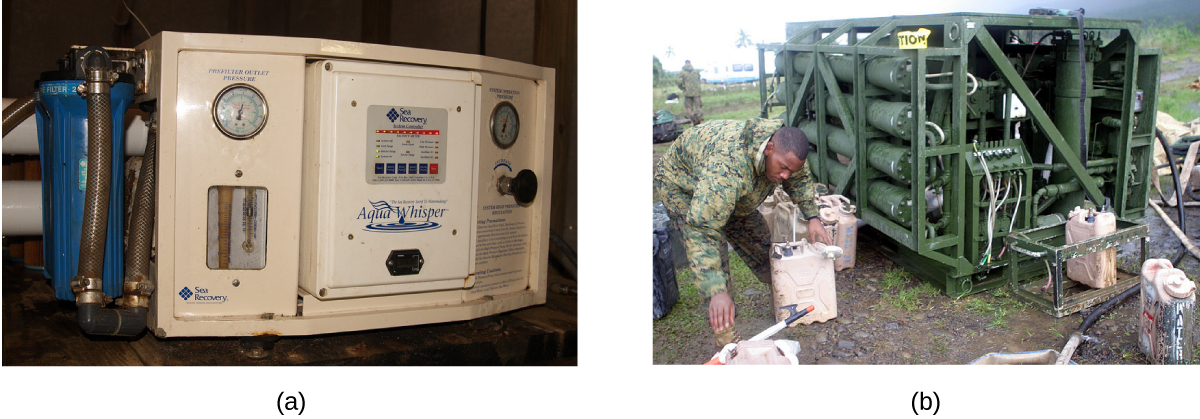Examples of osmosis are evident in many biological systems because cells are surrounded by semipermeable membranes. Carrots and celery that have become limp because they have lost water can be made crisp again by placing them in water. Water moves into the carrot or celery cells by osmosis. A cucumber placed in a concentrated salt solution loses water by osmosis and absorbs some salt to become a pickle. Osmosis can also affect animal cells. Solute concentrations are particularly important when solutions are injected into the body. Solutes in body cell fluids and blood serum give these solutions an osmotic pressure of approximately 7.7 atm. Solutions injected into the body must have the same osmotic pressure as blood serum; that is, they should be isotonic with blood serum. If a less concentrated solution, a hypotonic solution, is injected in sufficient quantity to dilute the blood serum, water from the diluted serum passes into the blood cells by osmosis, causing the cells to expand and rupture. This process is called hemolysis. When a more concentrated solution, a hypertonic solution, is injected, the cells lose water to the more concentrated solution, shrivel, and possibly die in a process called crenation. These effects are illustrated in (Figure). Red blood cell membranes are water permeable and will (a) swell and possibly rupture in a hypotonic solution; (b) maintain normal volume and shape in an isotonic solution; and (c) shrivel and possibly die in a hypertonic solution. (credit a/b/c: modifications of work by “LadyofHats”/Wikimedia commons)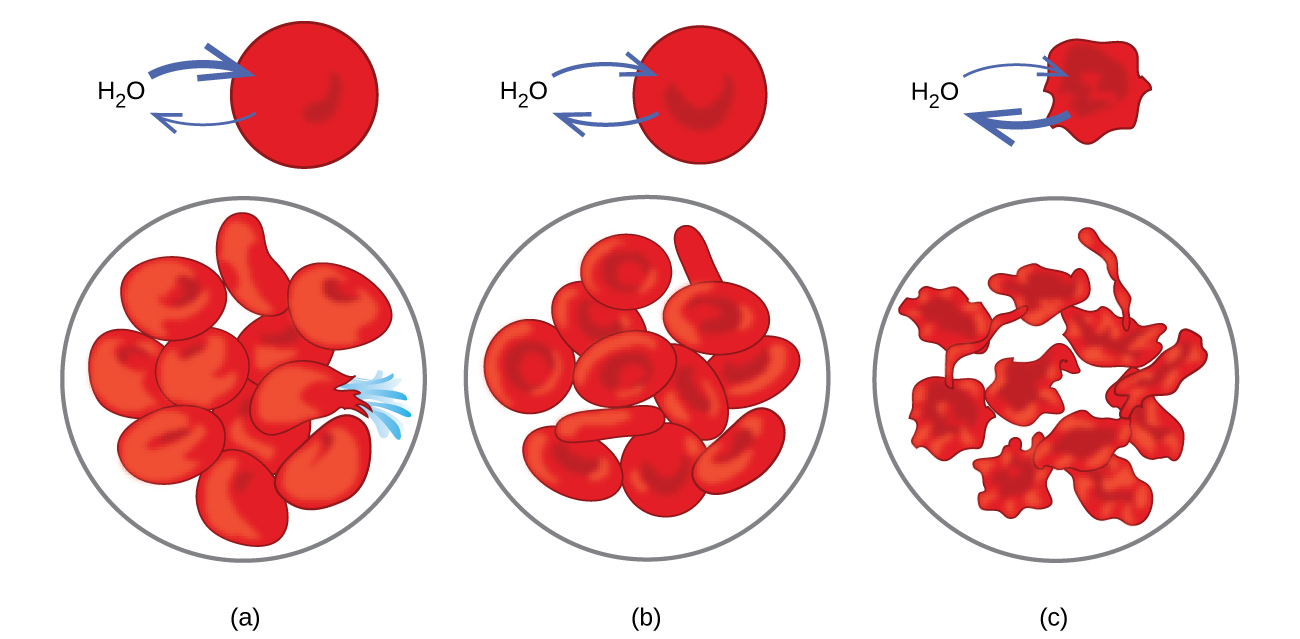### Determination of Molar Masses Osmotic pressure and changes in freezing point, boiling point, and vapor pressure are directly proportional to the number of solute species present in a given amount of solution. Consequently, measuring one of these properties for a solution prepared using a known mass of solute permits determination of the solute’s molar mass. Determination of a Molar Mass from a Freezing Point Depression A solution of 4.00 g of a nonelectrolyte dissolved in 55.0 g of benzene is found to freeze at 2.32 °C. Assuming ideal solution behavior, what is the molar mass of this compound? Solution Solve this problem using the following steps.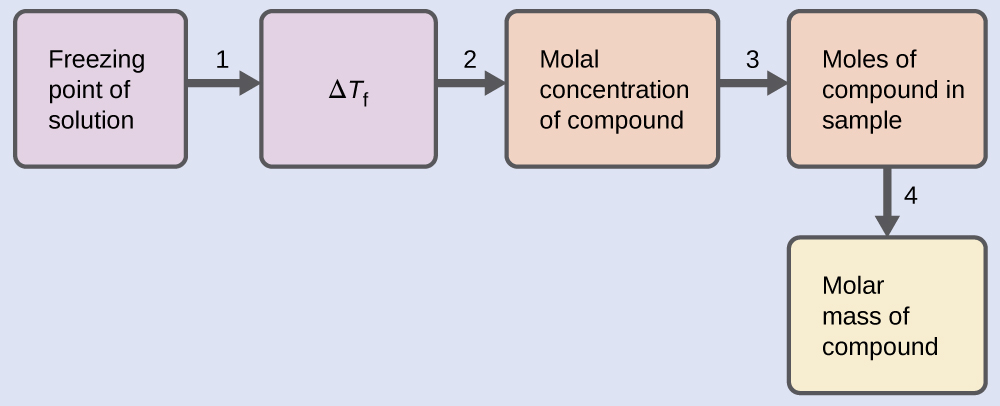1. Determine the change in freezing point from the observed freezing point and the freezing point of pure benzene ((Figure)).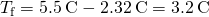2. Determine the molal concentration from Kf, the freezing point depression constant for benzene ((Figure)), and ΔTf.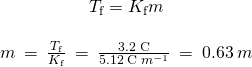3. Determine the number of moles of compound in the solution from the molal concentration and the mass of solvent used to make the solution.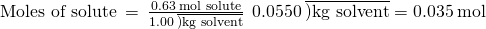4. Determine the molar mass from the mass of the solute and the number of moles in that mass.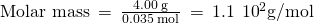Check Your Learning A solution of 35.7 g of a nonelectrolyte in 220.0 g of chloroform has a boiling point of 64.5 °C. Assuming ideal solution behavior, what is the molar mass of this compound? Answer: 1.8102 g/mol Determination of a Molar Mass from Osmotic Pressure A 0.500 L sample of an aqueous solution containing 10.0 g of hemoglobin has an osmotic pressure of 5.9 torr at 22 °C. Assuming ideal solution behavior, what is the molar mass of hemoglobin? Solution Here is one set of steps that can be used to solve the problem: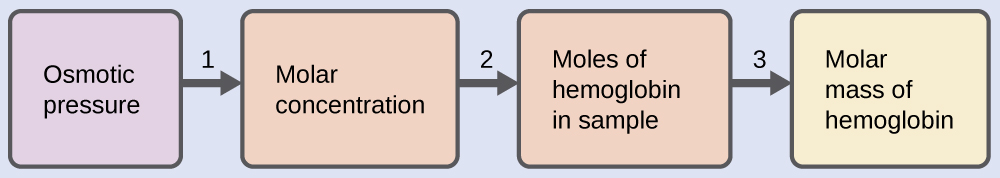1. Convert the osmotic pressure to atmospheres, then determine the molar concentration from the osmotic pressure. *** QuickLaTeX cannot compile formula: \begin{array}{}\\ \Pi \phantom{\rule{0.2em}{0ex}}=\phantom{\rule{0.2em}{0ex}}\frac{5.9\phantom{\rule{0.2em}{0ex}}\text{torr}\phantom{\rule{0.2em}{0ex}}×\phantom{\rule{0.2em}{0ex}}1\phantom{\rule{0.2em}{0ex}}\text{atm}}{760\phantom{\rule{0.2em}{0ex}}\text{torr}}\phantom{\rule{0.2em}{0ex}}=\phantom{\rule{0.2em}{0ex}}7.8\phantom{\rule{0.2em}{0ex}}×\phantom{\rule{0.2em}{0ex}}{10}^{-3}\text{atm}\\ \Pi =\mathit{\text{MRT}}\\ \\ M\phantom{\rule{0.2em}{0ex}}=\phantom{\rule{0.2em}{0ex}}\frac{\Pi }{RT}\phantom{\rule{0.2em}{0ex}}=\phantom{\rule{0.2em}{0ex}}\frac{7.8\phantom{\rule{0.2em}{0ex}}×\phantom{\rule{0.2em}{0ex}}{10}^{-3}\phantom{\rule{0.2em}{0ex}}\text{atm}}{\left(0.08206\phantom{\rule{0.2em}{0ex}}\text{L atm/mol K}\right)\left(295\phantom{\rule{0.2em}{0ex}}\text{K}\right)}\phantom{\rule{0.2em}{0ex}}=\phantom{\rule{0.2em}{0ex}}3.2\phantom{\rule{0.2em}{0ex}}×\phantom{\rule{0.2em}{0ex}}{10}^{-4}\text{M}\end{array} *** Error message: Missing # inserted in alignment preamble. leading text:\begin{array}{}
Missing $inserted. leading text:$\begin{array}{}\\ \Pi
Package inputenc Error: Unicode character × (U+00D7)
leading text: ...{760\phantom{\rule{0.2em}{0ex}}\text{torr}}
Package inputenc Error: Unicode character × (U+00D7)
leading text: ....2em}{0ex}}7.8\phantom{\rule{0.2em}{0ex}}×
Missing $inserted. leading text: ...\rule{0.2em}{0ex}}{10}^{-3}\text{atm}\\ \Pi Missing$ inserted.
leading text: ...\rule{0.2em}{0ex}}{10}^{-3}\text{atm}\\ \Pi
Missing $inserted. leading text: ...}\text{atm}\\ \Pi =\mathit{\text{MRT}}\\ \\ Missing$ inserted.
leading text: ...=\phantom{\rule{0.2em}{0ex}}\frac{\Pi }{RT}
Missing \$ inserted.
leading text: ...=\phantom{\rule{0.2em}{0ex}}\frac{\Pi }{RT}


2. Determine the number of moles of hemoglobin in the solution from the concentration and the volume of the solution.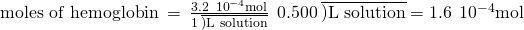3. Determine the molar mass from the mass of hemoglobin and the number of moles in that mass.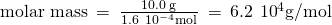Check Your Learning Assuming ideal solution behavior, what is the molar mass of a protein if a solution of 0.02 g of the protein in 25.0 mL of solution has an osmotic pressure of 0.56 torr at 25 °C?

Answer:

3104 g/mol

### Colligative Properties of Electrolytes

As noted previously in this module, the colligative properties of a solution depend only on the number, not on the identity, of solute species dissolved. The concentration terms in the equations for various colligative properties (freezing point depression, boiling point elevation, osmotic pressure) pertain to all solute species present in the solution. For the solutions considered thus far in this chapter, the solutes have been nonelectrolytes that dissolve physically without dissociation or any other accompanying process. Each molecule that dissolves yields one dissolved solute molecule. The dissolution of an electroyte, however, is not this simple, as illustrated by the two common examples below: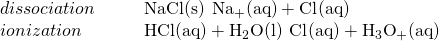Considering the first of these examples, and assuming complete dissociation, a 1.0 m aqueous solution of NaCl contains 2.0 mole of ions (1.0 mol Na+ and 1.0 mol Cl) per each kilogram of water, and its freezing point depression is expected to be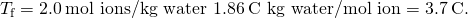When this solution is actually prepared and its freezing point depression measured, however, a value of 3.4 °C is obtained. Similar discrepancies are observed for other ionic compounds, and the differences between the measured and expected colligative property values typically become more significant as solute concentrations increase. These observations suggest that the ions of sodium chloride (and other strong electrolytes) are not completely dissociated in solution.

To account for this and avoid the errors accompanying the assumption of total dissociation, an experimentally measured parameter named in honor of Nobel Prize-winning German chemist Jacobus Henricus van’t Hoff is used. The van’t Hoff factor (i) is defined as the ratio of solute particles in solution to the number of formula units dissolved: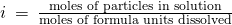Values for measured van’t Hoff factors for several solutes, along with predicted values assuming complete dissociation, are shown in (Figure).

Predicated and Measured van’t Hoff Factors for Several 0.050 m Aqueous Solutions
Formula unit Classification Dissolution products i (predicted) i (measured)
C12H22O11 (glucose) Nonelectrolyte C12H22O11 1 1.0
NaCl Strong electrolyte Na+, Cl 2 1.9
HCl Strong electrolyte (acid) H3O+, Cl 2 1.9
MgSO4 Strong electrolyte Mg2+, SO42−, 2 1.3
MgCl2 Strong electrolyte Mg2+, 2Cl 3 2.7
FeCl3 Strong electrolyte Fe3+, 3Cl 4 3.4

In 1923, the chemists Peter Debye and Erich Hückel proposed a theory to explain the apparent incomplete ionization of strong electrolytes. They suggested that although interionic attraction in an aqueous solution is very greatly reduced by solvation of the ions and the insulating action of the polar solvent, it is not completely nullified. The residual attractions prevent the ions from behaving as totally independent particles ((Figure)). In some cases, a positive and negative ion may actually touch, giving a solvated unit called an ion pair. Thus, the activity, or the effective concentration, of any particular kind of ion is less than that indicated by the actual concentration. Ions become more and more widely separated the more dilute the solution, and the residual interionic attractions become less and less. Thus, in extremely dilute solutions, the effective concentrations of the ions (their activities) are essentially equal to the actual concentrations. Note that the van’t Hoff factors for the electrolytes in (Figure) are for 0.05 m solutions, at which concentration the value of i for NaCl is 1.9, as opposed to an ideal value of 2.

Dissociation of ionic compounds in water is not always complete due to the formation of ion pairs.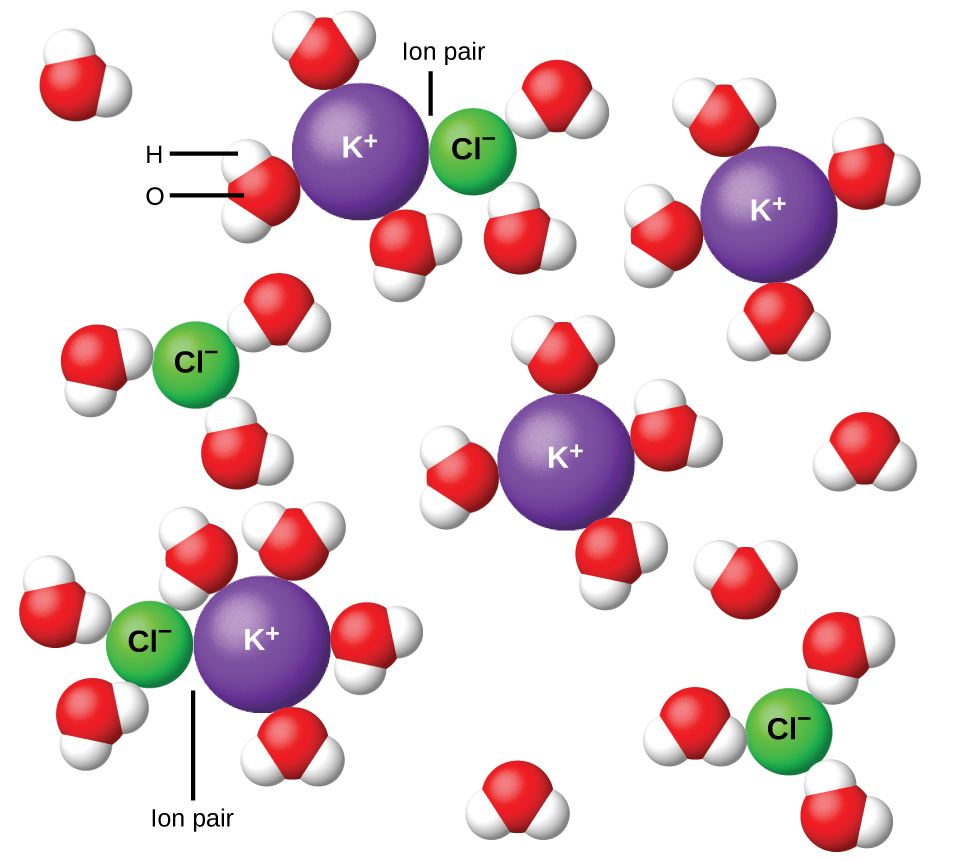The Freezing Point of a Solution of an Electrolyte The concentration of ions in seawater is approximately the same as that in a solution containing 4.2 g of NaCl dissolved in 125 g of water. Use this information and a predicted value for the van’t Hoff factor ((Figure)) to determine the freezing temperature the solution (assume ideal solution behavior).

Solution Solve this problem using the following series of steps.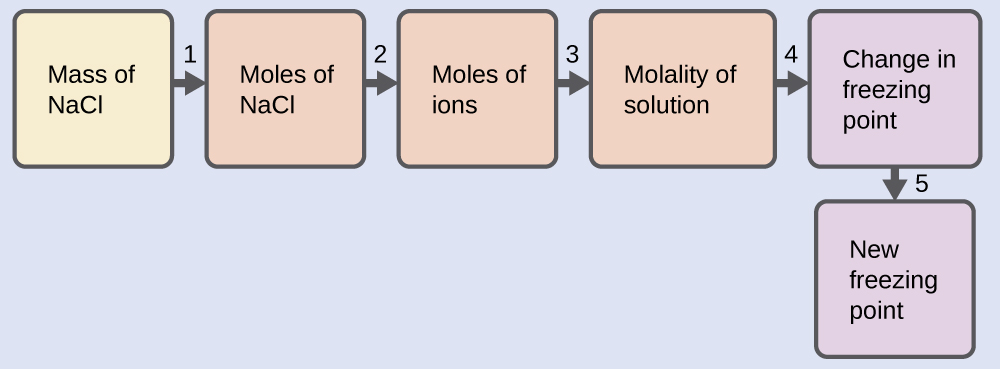1. Convert from grams to moles of NaCl using the molar mass of NaCl in the unit conversion factor.
Result: 0.072 mol NaCl
2. Determine the number of moles of ions present in the solution using the number of moles of ions in 1 mole of NaCl as the conversion factor (2 mol ions/1 mol NaCl).
Result: 0.14 mol ions
3. Determine the molality of the ions in the solution from the number of moles of ions and the mass of solvent, in kilograms.
Result: 1.2 m
4. Use the direct proportionality between the change in freezing point and molal concentration to determine how much the freezing point changes.
Result: 2.1 °C
5. Determine the new freezing point from the freezing point of the pure solvent and the change.
Result: −2.1 °C
Check each result as a self-assessment, taking care to avoid rounding errors by retaining guard digits in each step’s result for computing the next step’s result.

Check Your Learning Assuming complete dissociation and ideal solution behavior, calculate the freezing point of a solution of 0.724 g of CaCl2 in 175 g of water.

Answer:

−0.208 °C

### Key Concepts and Summary

Properties of a solution that depend only on the concentration of solute particles are called colligative properties. They include changes in the vapor pressure, boiling point, and freezing point of the solvent in the solution. The magnitudes of these properties depend only on the total concentration of solute particles in solution, not on the type of particles. The total concentration of solute particles in a solution also determines its osmotic pressure. This is the pressure that must be applied to the solution to prevent diffusion of molecules of pure solvent through a semipermeable membrane into the solution. Ionic compounds may not completely dissociate in solution due to activity effects, in which case observed colligative effects may be less than predicted.

### Key Equations

•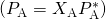•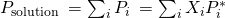•• ΔTb = Kbm
• ΔTf = Kfm
• Π = MRT

### Chemistry End of Chapter Exercises

Which is/are part of the macroscopic domain of solutions and which is/are part of the microscopic domain: boiling point elevation, Henry’s law, hydrogen bond, ion-dipole attraction, molarity, nonelectrolyte, nonstoichiometric compound, osmosis, solvated ion?

What is the microscopic explanation for the macroscopic behavior illustrated in (Figure)?

The strength of the bonds between like molecules is stronger than the strength between unlike molecules. Therefore, some regions will exist in which the water molecules will exclude oil molecules and other regions will exist in which oil molecules will exclude water molecules, forming a heterogeneous region.

Sketch a qualitative graph of the pressure versus time for water vapor above a sample of pure water and a sugar solution, as the liquids evaporate to half their original volume.

A solution of potassium nitrate, an electrolyte, and a solution of glycerin (C3H5(OH)3), a nonelectrolyte, both boil at 100.3 °C. What other physical properties of the two solutions are identical?

Both form homogeneous solutions; their boiling point elevations are the same, as are their lowering of vapor pressures. Osmotic pressure and the lowering of the freezing point are also the same for both solutions.

What are the mole fractions of H3PO4 and water in a solution of 14.5 g of H3PO4 in 125 g of water?

(a) Outline the steps necessary to answer the question.

(b) Answer the question.

What are the mole fractions of HNO3 and water in a concentrated solution of nitric acid (68.0% HNO3 by mass)?

(a) Outline the steps necessary to answer the question.

(b) Answer the question.

(a) Find number of moles of HNO3 and H2O in 100 g of the solution. Find the mole fractions for the components. (b) The mole fraction of HNO3 is 0.378. The mole fraction of H2O is 0.622.

Calculate the mole fraction of each solute and solvent:

(a) 583 g of H2SO4 in 1.50 kg of water—the acid solution used in an automobile battery

(b) 0.86 g of NaCl in 1.00102 g of water—a solution of sodium chloride for intravenous injection

(c) 46.85 g of codeine, C18H21NO3, in 125.5 g of ethanol, C2H5OH

(d) 25 g of I2 in 125 g of ethanol, C2H5OH

Calculate the mole fraction of each solute and solvent:

(a) 0.710 kg of sodium carbonate (washing soda), Na2CO3, in 10.0 kg of water—a saturated solution at 0 °C

(b) 125 g of NH4NO3 in 275 g of water—a mixture used to make an instant ice pack

(c) 25 g of Cl2 in 125 g of dichloromethane, CH2Cl2

(d) 0.372 g of tetrahydropyridine, C5H9N, in 125 g of chloroform, CHCl3

(a)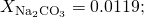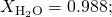(b)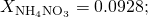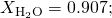(c)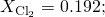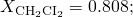(d)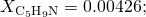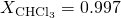Calculate the mole fractions of methanol, CH3OH; ethanol, C2H5OH; and water in a solution that is 40% methanol, 40% ethanol, and 20% water by mass. (Assume the data are good to two significant figures.)

What is the difference between a 1 M solution and a 1 m solution?

In a 1 M solution, the mole is contained in exactly 1 L of solution. In a 1 m solution, the mole is contained in exactly 1 kg of solvent.

What is the molality of phosphoric acid, H3PO4, in a solution of 14.5 g of H3PO4 in 125 g of water?

(a) Outline the steps necessary to answer the question.

(b) Answer the question.

What is the molality of nitric acid in a concentrated solution of nitric acid (68.0% HNO3 by mass)?

(a) Outline the steps necessary to answer the question.

(b) Answer the question.

(a) Determine the molar mass of HNO3. Determine the number of moles of acid in the solution. From the number of moles and the mass of solvent, determine the molality. (b) 33.7 m

Calculate the molality of each of the following solutions:

(a) 583 g of H2SO4 in 1.50 kg of water—the acid solution used in an automobile battery

(b) 0.86 g of NaCl in 1.00102 g of water—a solution of sodium chloride for intravenous injection

(c) 46.85 g of codeine, C18H21NO3, in 125.5 g of ethanol, C2H5OH

(d) 25 g of I2 in 125 g of ethanol, C2H5OH

Calculate the molality of each of the following solutions:

(a) 0.710 kg of sodium carbonate (washing soda), Na2CO3, in 10.0 kg of water—a saturated solution at 0°C

(b) 125 g of NH4NO3 in 275 g of water—a mixture used to make an instant ice pack

(c) 25 g of Cl2 in 125 g of dichloromethane, CH2Cl2

(d) 0.372 g of tetrahydropyridine, C5H9N, in 125 g of chloroform, CHCl3

(a) 6.7010−1m; (b) 5.67 m; (c) 2.8 m; (d) 0.0358 m

The concentration of glucose, C6H12O6, in normal spinal fluid is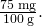What is the molality of the solution?

A 13.0% solution of K2CO3 by mass has a density of 1.09 g/cm3. Calculate the molality of the solution.

1.08 m

Why does 1 mol of sodium chloride depress the freezing point of 1 kg of water almost twice as much as 1 mol of glycerin?

Assuming ideal solution behavior, what is the boiling point of a solution of 115.0 g of nonvolatile sucrose, C12H22O11, in 350.0 g of water?

(a) Outline the steps necessary to answer the question

(b) Answer the question

(a) Determine the molar mass of sucrose; determine the number of moles of sucrose in the solution; convert the mass of solvent to units of kilograms; from the number of moles and the mass of solvent, determine the molality; determine the difference between the boiling point of water and the boiling point of the solution; determine the new boiling point. (b) 100.5 °C

Assuming ideal solution behavior, what is the boiling point of a solution of 9.04 g of I2 in 75.5 g of benzene, assuming the I2 is nonvolatile?

(a) Outline the steps necessary to answer the question.

(b) Answer the question.

Assuming ideal solution behavior, what is the freezing temperature of a solution of 115.0 g of sucrose, C12H22O11, in 350.0 g of water?

(a) Outline the steps necessary to answer the question.

(b) Answer the question.

(a) Determine the molar mass of sucrose; determine the number of moles of sucrose in the solution; convert the mass of solvent to units of kilograms; from the number of moles and the mass of solvent, determine the molality; determine the difference between the freezing temperature of water and the freezing temperature of the solution; determine the new freezing temperature. (b) −1.8 °C

Assuming ideal solution behavior, what is the freezing point of a solution of 9.04 g of I2 in 75.5 g of benzene?

(a) Outline the steps necessary to answer the following question.

(b) Answer the question.

Assuming ideal solution behavior, what is the osmotic pressure of an aqueous solution of 1.64 g of Ca(NO3)2 in water at 25 °C? The volume of the solution is 275 mL.

(a) Outline the steps necessary to answer the question.

(b) Answer the question.

(a) Determine the molar mass of Ca(NO3)2; determine the number of moles of Ca(NO3)2 in the solution; determine the number of moles of ions in the solution; determine the molarity of ions, then the osmotic pressure. (b) 2.67 atm

Assuming ideal solution behavior, what is osmotic pressure of a solution of bovine insulin (molar mass, 5700 g mol−1) at 18 °C if 100.0 mL of the solution contains 0.103 g of the insulin?

(a) Outline the steps necessary to answer the question.

(b) Answer the question.

Assuming ideal solution behavior, what is the molar mass of a solution of 5.00 g of a compound in 25.00 g of carbon tetrachloride (bp 76.8 °C; Kb = 5.02 °C/m) that boils at 81.5 °C at 1 atm?

(a) Outline the steps necessary to answer the question.

(b) Solve the problem.

(a) Determine the molal concentration from the change in boiling point and Kb; determine the moles of solute in the solution from the molal concentration and mass of solvent; determine the molar mass from the number of moles and the mass of solute. (b) 2.1102 g mol−1

A sample of an organic compound (a nonelectrolyte) weighing 1.35 g lowered the freezing point of 10.0 g of benzene by 3.66 °C. Assuming ideal solution behavior, calculate the molar mass of the compound.

A 1.0 m solution of HCl in benzene has a freezing point of 0.4 °C. Is HCl an electrolyte in benzene? Explain.

No. Pure benzene freezes at 5.5 °C, and so the observed freezing point of this solution is depressed by ΔTf = 5.5 − 0.4 = 5.1 °C. The value computed, assuming no ionization of HCl, is ΔTf = (1.0 m)(5.14 °C/m) = 5.1 °C. Agreement of these values supports the assumption that HCl is not ionized.

A solution contains 5.00 g of urea, CO(NH2)2, a nonvolatile compound, dissolved in 0.100 kg of water. If the vapor pressure of pure water at 25 °C is 23.7 torr, what is the vapor pressure of the solution (assuming ideal solution behavior)?

A 12.0-g sample of a nonelectrolyte is dissolved in 80.0 g of water. The solution freezes at −1.94 °C. Assuming ideal solution behavior, calculate the molar mass of the substance.

144 g mol−1

Arrange the following solutions in order by their decreasing freezing points: 0.1 m Na3PO4, 0.1 m C2H5OH, 0.01 m CO2, 0.15 m NaCl, and 0.2 m CaCl2.

Calculate the boiling point elevation of 0.100 kg of water containing 0.010 mol of NaCl, 0.020 mol of Na2SO4, and 0.030 mol of MgCl2, assuming complete dissociation of these electrolytes and ideal solution behavior.

0.870 °C

How could you prepare a 3.08 m aqueous solution of glycerin, C3H8O3? Assuming ideal solution behavior, what is the freezing point of this solution?

A sample of sulfur weighing 0.210 g was dissolved in 17.8 g of carbon disulfide, CS2 (Kb = 2.43 °C/m). If the boiling point elevation was 0.107 °C, what is the formula of a sulfur molecule in carbon disulfide (assuming ideal solution behavior)?

S8

In a significant experiment performed many years ago, 5.6977 g of cadmium iodide in 44.69 g of water raised the boiling point 0.181 °C. What does this suggest about the nature of a solution of CdI2?

Lysozyme is an enzyme that cleaves cell walls. A 0.100-L sample of a solution of lysozyme that contains 0.0750 g of the enzyme exhibits an osmotic pressure of 1.3210−3 atm at 25 °C. Assuming ideal solution behavior, what is the molar mass of lysozyme?

1.39104 g mol−1

The osmotic pressure of a solution containing 7.0 g of insulin per liter is 23 torr at 25 °C. Assuming ideal solution behavior, what is the molar mass of insulin?

The osmotic pressure of human blood is 7.6 atm at 37 °C. What mass of glucose, C6H12O6, is required to make 1.00 L of aqueous solution for intravenous feeding if the solution must have the same osmotic pressure as blood at body temperature, 37 °C (assuming ideal solution behavior)?

54 g

Assuming ideal solution behavior, what is the freezing point of a solution of dibromobenzene, C6H4Br2, in 0.250 kg of benzene, if the solution boils at 83.5 °C?

Assuming ideal solution behavior, what is the boiling point of a solution of NaCl in water if the solution freezes at −0.93 °C?

100.26 °C

The sugar fructose contains 40.0% C, 6.7% H, and 53.3% O by mass. A solution of 11.7 g of fructose in 325 g of ethanol has a boiling point of 78.59 °C. The boiling point of ethanol is 78.35 °C, and Kb for ethanol is 1.20 °C/m. Assuming ideal solution behavior, what is the molecular formula of fructose?

The vapor pressure of methanol, CH3OH, is 94 torr at 20 °C. The vapor pressure of ethanol, C2H5OH, is 44 torr at the same temperature.

(a) Calculate the mole fraction of methanol and of ethanol in a solution of 50.0 g of methanol and 50.0 g of ethanol.

(b) Ethanol and methanol form a solution that behaves like an ideal solution. Calculate the vapor pressure of methanol and of ethanol above the solution at 20 °C.

(c) Calculate the mole fraction of methanol and of ethanol in the vapor above the solution.

(a)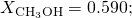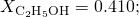(b) Vapor pressures are: CH3OH: 55 torr; C2H5OH: 18 torr; (c) CH3OH: 0.75; C2H5OH: 0.25

The triple point of air-free water is defined as 273.16 K. Why is it important that the water be free of air?

Meat can be classified as fresh (not frozen) even though it is stored at −1 °C. Why wouldn’t meat freeze at this temperature?

The ions and compounds present in the water in the beef lower the freezing point of the beef below −1 °C.

An organic compound has a composition of 93.46% C and 6.54% H by mass. A solution of 0.090 g of this compound in 1.10 g of camphor melts at 158.4 °C. The melting point of pure camphor is 178.4 °C. Kf for camphor is 37.7 °C/m. Assuming ideal solution behavior, what is the molecular formula of the solute? Show your calculations.

A sample of HgCl2 weighing 9.41 g is dissolved in 32.75 g of ethanol, C2H5OH (Kb = 1.20 °C/m). The boiling point elevation of the solution is 1.27 °C. Is HgCl2 an electrolyte in ethanol? Show your calculations.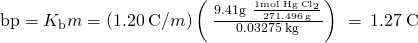The observed change equals the theoretical change; therefore, no dissociation occurs.

A salt is known to be an alkali metal fluoride. A quick approximate determination of freezing point indicates that 4 g of the salt dissolved in 100 g of water produces a solution that freezes at about −1.4 °C. Assuming ideal solution behavior, what is the formula of the salt? Show your calculations.

### Glossary

boiling point elevation
elevation of the boiling point of a liquid by addition of a solute
boiling point elevation constant
the proportionality constant in the equation relating boiling point elevation to solute molality; also known as the ebullioscopic constant
colligative property
property of a solution that depends only on the concentration of a solute species
crenation
process whereby biological cells become shriveled due to loss of water by osmosis
freezing point depression
lowering of the freezing point of a liquid by addition of a solute
freezing point depression constant
(also, cryoscopic constant) proportionality constant in the equation relating freezing point depression to solute molality
hemolysis
rupture of red blood cells due to the accumulation of excess water by osmosis
hypertonic
of greater osmotic pressure
hypotonic
of less osmotic pressure
ion pair
solvated anion/cation pair held together by moderate electrostatic attraction
isotonic
of equal osmotic pressure
molality (m)
a concentration unit defined as the ratio of the numbers of moles of solute to the mass of the solvent in kilograms
osmosis
diffusion of solvent molecules through a semipermeable membrane
osmotic pressure (Π)
opposing pressure required to prevent bulk transfer of solvent molecules through a semipermeable membrane
Raoult’s law
the relationship between a solution’s vapor pressure and the vapor pressures and concentrations of its components
semipermeable membrane
a membrane that selectively permits passage of certain ions or molecules
van’t Hoff factor (i)
the ratio of the number of moles of particles in a solution to the number of moles of formula units dissolved in the solution

## LicenseChemistry 2e by OSCRiceUniversity is licensed under a Creative Commons Attribution 4.0 International License, except where otherwise noted.